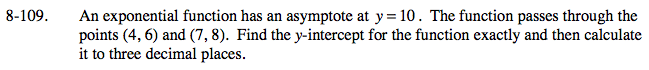### Home > PC > Chapter 8 > Lesson 8.2.3 > Problem8-109

8-109.Write the exponential form:

Substitute each point in for (x, y) forming two equations and two unknowns.

y = (a)bx + 10

6 = (a)(b)4 + 10
8 = (a)(b)7 + 10

Solve for 'a' and 'b' and substitute the contants into the function.

Using the new function, substitute '0' for 'x' and simplify. This is the y-intercept.